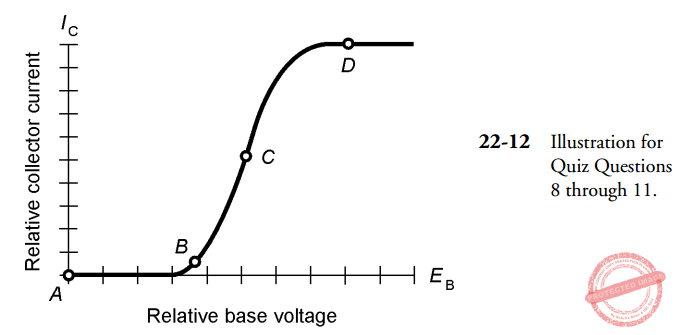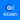# Gibilisco: MCQ in The Bipolar Transistor | ECE Board Exam

(Last Updated On: March 16, 2020)This is the Multiple Choice Questions (MCQs) in Chapter 22: Bipolar Transistor from the book Teach Yourself Electricity and Electronics, 5th edition by Stan Gibilisco. If you are looking for a reviewer in Electronics Engineering this will definitely help you before taking the Board Exam.

### Start Practice Exam Test Questions

1. In a PNP circuit, the collector:

• A. Has an arrow pointing inward.
• B. Is positive with respect to the emitter.
• C. Is biased at a small fraction of the base bias.
• D. Is negative with respect to the emitter.

2. In many cases, a PNP transistor can be replaced with an NPN device and the circuit will do the same thing, provided that:

• A. The supply polarity is reversed.
• B. The collector and emitter leads are interchanged.
• C. The arrow is pointing inward.
• D. No! A PNP device cannot be replaced with an NPN.

3. A bipolar transistor has:

• A. Three P-N junctions.
• B. Three semiconductor layers.
• C. Two N-type layers around a P-type layer.
• D. A low avalanche voltage.

4. In the dual-diode model of an NPN transistor, the emitter corresponds to:

• A. The point where the cathodes are connected together.
• B. The point where the cathode of one diode is connected to the anode of the other.
• C. The point where the anodes are connected together.
• D. Either of the diode cathodes.

5. The current through a transistor depends on:

• A. EC.
• B. EB relative to EC.
• C. IB.
• D. More than one of the above.

6. With no signal input, a bipolar transistor would have the least IC when:

• A. The emitter is grounded.
• B. The E-B junction is forward biased.
• C. The E-B junction is reverse biased.
• D. The E-B current is high.

7. When a transistor is conducting as much as it possibly can, it is said to be:

• A. In cutoff.
• B. In saturation.
• C. Forward biased.
• D. In avalanche.

NOTE: For questions 8 – 11 see Fig.22-12.8. Refer to Fig. 22-12. The best point at which to operate a transistor as a small-signal amplifier is:

• A. A.
• B. B.
• C. C.
• D. D.

9. In Fig. 22-12, the forward-breakover point for the E-B junction is nearest to:

• A. No point on this graph.
• B. B.
• C. C.
• D. D.

10. In Fig. 22-12, saturation is nearest to point:

• A. A.
• B. B.
• C. C.
• D. D.

11. In Fig. 22-12, the greatest gain occurs at point:

• A. A.
• B. B.
• C. C.
• D. D.

12. In a common-emitter circuit, the gain bandwidth product is:

• A. The frequency at which the gain is 1.
• B. The frequency at which the gain is 0.707 times its value at 1 MHz.
• C. The frequency at which the gain is greatest.
• D. The difference between the frequency at which the gain is greatest, and the frequency at which the gain is 1.

13. The configuration most often used for matching a high input impedance to a low output impedance puts signal ground at:

• A. The emitter.
• B. The base.
• C. The collector.
• D. Any point; it doesn’t matter.

14. The output is in phase with the input in a:

• A. Common-emitter circuit.
• B. Common-base circuit.
• C. Common-collector circuit.
• D. More than one of the above.

15. The greatest possible amplification is obtained in:

• A. A common-emitter circuit.
• B. A common-base circuit.
• C. A common-collector circuit.
• D. More than one of the above.

16. The input is applied to the collector in:

• A. A common-emitter circuit.
• B. A common-base circuit.
• C. A common-collector circuit.
• D. None of the above.

17. The configuration noted for its stability in radio-frequency power amplifiers is the:

• A. Common-emitter circuit.
• B. Common-base circuit.
• C. Common-collector circuit.
• D. Emitter-follower circuit.

18. In a common-base circuit, the output is taken from the:

• A. Emitter.
• B. Base.
• C. Collector.
• D. More than one of the above.

19. The input signal to a transistor amplifier results in saturation during part of the cycle. This produces:

• A. The greatest possible amplification.
• B. Reduced efficiency.
• C. Avalanche effect.
• D. Nonlinear output impedance.

20. The gain of a transistor in a common-emitter circuit is 100 at a frequency of 1000 Hz. The gain is 70.7 at 335 kHz. The gain drops to 1 at 210 MHz. The alpha cutoff is:

• A. 1 kHz.
• B. 335 kHz.
• C. 210 MHz.
• D. None of the above.

### Complete List of Multiple Choice Questions from this Book

Help Me Makes a Difference!

 P inoyBIX educates thousands of reviewers/students a day in preparation for their board examinations. Also provides professionals with materials for their lectures and practice exams. Help me go forward with the same spirit. “Will you make a small gift today via GCASH?”+63 966 459 6474 Option 1 : \$1 USD Option 2 : \$3 USD Option 3 : \$5 USD Option 4 : \$10 USD Option 5 : \$25 USD Option 6 : \$50 USD Option 7 : \$100 USD Option 8 : Other Amount© 2014 PinoyBIX Engineering. © 2019 All Rights Reserved | How to Donate? |